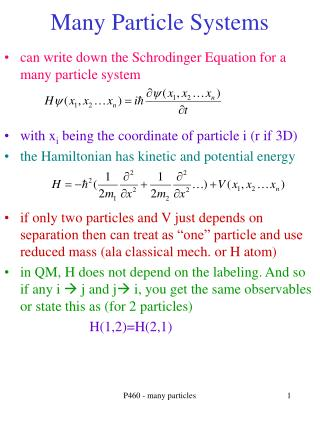DownloadDownload PresentationMany Particle Systems

# Many Particle Systems

Download Presentation## Many Particle Systems

- - - - - - - - - - - - - - - - - - - - - - - - - - - E N D - - - - - - - - - - - - - - - - - - - - - - - - - - -
##### Presentation Transcript

1. Many Particle Systems • can write down the Schrodinger Equation for a many particle system • with xi being the coordinate of particle i (r if 3D) • the Hamiltonian has kinetic and potential energy • if only two particles and V just depends on separation then can treat as “one” particle and use reduced mass (ala classical mech. or H atom) • in QM, H does not depend on the labeling. And so if any i  j and j i, you get the same observables or state this as (for 2 particles) H(1,2)=H(2,1) P460 - many particles

2. Exchange Operator • for now keep using 2 particle as example. Use 1,2 for both space coordinates and quantum states (like spin) • can formally define the exchange operator • as the eigenvalues of H do not depend on 1,2, implies that P12 is a constant of motion • can then define symmetric and antisymmetric states. If start out in an eigenstate, then stays in it at future times N = normalization. so for 2 and 3 particle systems P460 - many particles

3. Schrod. Eq. For 2 Particles • have kinetic energy term for both electrons (1+2) • let V12 be 0 for now (can still make sym/antisym in any case) • easy to show then that one can then separate variables and the wavefunction is: • where these are (identical) single particle wavefunctions (i.e. Hydrogen, infinite box) • define format. 1 (2) is particle 1’s (2’s) position and a,b are the quantum numbers for that eigenfunction P460 - many particles

4. Identical Particles A • Particles are represented by wave packets. If packet A has mass = .511 MeV, spin=1/2, charge= -1, then it is an electron • any wave packet with this feature is indistinguishable • can’t really tell the “blue” from the “magenta” packet after they overlap • an ensemble (1 or more) of spin ½,3/2...particles (Fermions) have antisymmetric wavefunctions while spin 0,1,2...particles (Bosons) are described by symmetric. Note also holds for 1 particle (remember rotating spin ½ by 360 degrees. gave phase of -1) T0 t1>t0 t2>t1 P460 - many particles

5. Identical Particles • Create wave function for 2 particles • the 2 ways of making the wavefunction are degenerate--they have the same energy--and can use any linear combination of the wavefunctions • Want to have a wavefunction whose probability (that is all measured quantities) is the same if 1 and 2 are “flipped” • These are NOT the same. Instead use linear combinations (as degenerate). Have a symmetric and an antisymmetric combination P460 - many particles

6. 2 Identical Particles in a Box • Create wave function for 2 particles in a box • the antisymmetric term = 0 if either both particles are in the same quantum state (Pauli exclusion) OR if x1 = x2 • suppression of ANTI when 2 particles are close to each other. Enhancement of SYM when two particles are close to each other • this gives different values for the average separation <|x2 –x1 |> and so different values for the added term in the energy that depend on particle separation (like e-e repulsion)….or different energy levels for the ANTI and SYM spatial wave functions (the degeneracy is broken) P460 - many particles

7. Particles in a Box and Spin S=1 • Have spatial wave function for 2 particles in a box which are either symmetric or antsymmetric • there is also the spin. assume s= ½ • as Fermions need totally antisymmetric: spatial Asymmetric + spin Symmetric (S=1) spatial symmetric + spin Antisymmetric (S=0) • if Boson, need totally symmetric and so symmetric*symmetric or antisymmetric*antisymmetric S=0 P460 - many particles

8. Multiparticle eigenstates • Need an antisymmetric wave function (1,2,3 are positions; i,j,k…are quantum states). Can make using determinant: • while it is properly antisymmetric for any 1j. For atoms, practically only need to worry about valence effects. Solids, different terms lead to energy bands P460 - many particles

9. Density of States • any system determine density of states D=dN/dE • can do for a gas of uninteracting but overlapping identical particles • density of states the same for Bosons or Fermions but how they are filled (the probability) and so average energy, etc will be different (quantum statistics – do in 461) • for Fermions (i.e. electrons), Pauli exclusion holds and so particles fill up lower states • at T=0 fill up states up to Fermi Energy EF. Fermi energy depends on density as gives total number of particles available for filling up states P460 - many particles

10. Density of States II • # of available states (“nodes”) for any wavelength • wavelength  momentum  energy • “standing wave” counting often holds:often called “gas” but can be solid/liquid. Solve Scrd. Eq. In 1D • go to 3D. ni>0 and look at 1/8 of sphere 0 L i.e. 2s+1 P460 - many particles

11. Density of States III • convert to momentum • convert to energy depends on kinematics relativistic • non-relativistic P460 - many particles

12. Density of States IV • can find Fermi energy (assume T=0). Find number of states (n) Nonrelativistic • compare to lowest energy of particle in a box of size a with l=2a (good way of remembering EF) • and this in some sense gives the condition on overlapping (particle indistinguishability). two identical particles in the same quantum state have P460 - many particles